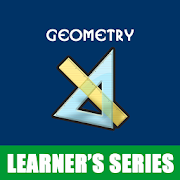## Geometry Mathematics - Lumos Educational App Store3.91
Price -FREE
\$0

#### DESCRIPTION:

This application for Geometry gives us clear idea about the concepts of Euclidean Geometry. Deals all about geometrical figures, expressions and other significant parameters. This application help the students of all Standards to learn all about Triangle, Rectangle, Square, Cube , Circle, Sphere, Hemisphere and Cone. Another important advantage about this application is, this clearly deals formulas to find the various geometrical parameters like Perimeter,Area, Volume and other important measurements of all geometrical figures.This explains Pythagorean theorem for Right angled triangle. we hop

#### OVERVIEW:

Geometry Mathematics is a free educational mobile app By Learner's Series.It helps students in grades 2 practice the following standards 2.G.A.1.

This page not only allows students and teachers download Geometry Mathematics but also find engaging Sample Questions, Videos, Pins, Worksheets, Books related to the following topics.

1. 2.G.A.1 : Recognize and draw shapes having specified attributes, such as a given number of angles or a given number of equal faces.

2

#### STANDARDS:

2.G.A.1

Developer: Learner's Series

Software Version: 1.5.3

Category: Education

### RELATED APPSEdSearch WebSearch